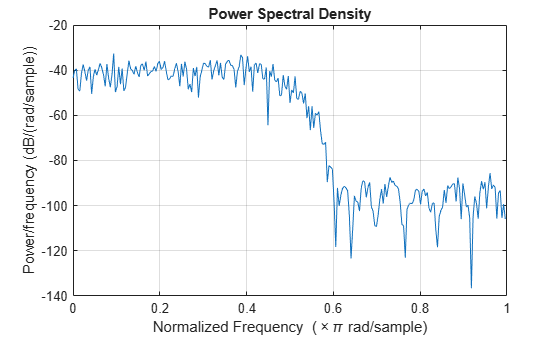# noisepsd

Power spectral density of filter output due to roundoff noise

## Syntax

```hpsd = noisepsd(sysobj,L) hpsd = noisepsd(sysobj,L,param1,value1,param2,value2,...) hpsd = noisepsd(sysobj,L,opts) noisepsd(sysobj,...) ```

## Description

`hpsd = noisepsd(sysobj,L)` computes the power spectral density (PSD) at the output of filter System object™, `sysobj`, occurring because of roundoff noise. This noise is produced by quantization errors within the filter. `L` is the number of trials used to compute the average. The PSD is computed from the average over the `L` trials. The more trials you specify, the better the estimate, but at the expense of longer computation time. When you do not explicitly specify `L`, the default is 10 trials.

`hpsd` is a `psd` data object. To extract the PSD vector (the data from the PSD) from `hpsd`, enter

`get(hpsd,'data')`

at the prompt. Plot the PSD data with `plot(hpsd)`. The average power of the output noise (the integral of the PSD) can be computed with `avgpower`, a method of `dspdata` objects:

`avgpwr = avgpower(hpsd).`

`hpsd = noisepsd(sysobj,L,param1,value1,param2,value2,...)` where `sysobj` is a filter System object, specifies optional parameters via propertyname/propertyvalue pairs. Valid `psd` object property values are:

Property Name

Default Value

Description and Valid Entries

`Nfft`

`512`

Specify the number of FFT points to use to calculate the PSD.

`NormalizedFrequency`

`true`

Determine whether to use normalized frequency. Enter a logical value of `true` or `false`. Because this property is a logical value, do not enclose the single quotation marks.

`Fs`

`normalized`

Specify the sampling frequency to use when you set `NormalizedFrequency` to `false`. Use any integer value greater than 1. Enter the value in Hz.

`SpectrumType`

`onesided`

Specify how `noisepsd` should generate the PSD. Options are `onesided` or `twosided`. If you choose a two-sided computation, you can also choose `CenterDC` = `true`. Otherwise, `CenterDC` must be `false`.

• `onesided` converts the type to a spectrum that is calculated over half the Nyquist interval. All properties affected by the new frequency range are adjusted automatically.

• `twosided` converts the type to a spectrum that is calculated over the whole Nyquist interval. All properties affected by the new frequency range are adjusted automatically.

`CenterDC`

`false`

Shift the zero-frequency component to the center of a two-sided spectrum.

• When you set `SpectrumType` to `onesided`, it is changed to `twosided` and the data is converted to a two-sided spectrum.

• Setting `CenterDC` to `false` shifts the data and the frequency values in the object so that DC is in the left edge of the spectrum. This operation does not affect the `SpectrumType` property setting.

`Arithmetic``ARITH`Analyze the filter System object, based on the arithmetic specified in the `ARITH` input. `ARITH` can be set to `double`, `single`, or `fixed`. The analysis tool assumes a double-precision filter when the arithmetic input is not specified and the filter System object is in an unlocked state.

### Note

If the spectrum data you specify is calculated over half the Nyquist interval and you do not specify a corresponding frequency vector, the default frequency vector assumes that the number of points in the whole FFT was even. Also, the plot option to convert to a whole or two-sided spectrum assumes the original whole FFT length is even.

`noisepsd` requires knowledge of the input data type. Analysis cannot be performed if the input data type is not available. If you do not specify the `Arithmetic` parameter, i.e., use the syntax `[h,w] = noisepsd(sysobj)` , then the following rules apply to this method:

• The System object state is `Unlocked``noisepsd` performs double-precision analysis.

• The System object state is `Locked``noisepsd` performs analysis based on the locked input data type.

If you do specify the `Arithmetic` parameter, i.e., use the syntax ```[h,w] = noisepsd(sysobj,'Arithmetic', ARITH)```, review the following rules for this method. Which rule applies depends on the value you set for the `Arithmetic` parameter.

`Value`System Object StateRule
`ARITH = 'double'``Unlocked``noisepsd` performs double-precision analysis.
`Locked``noisepsd` performs double-precision analysis.
`ARITH = 'single'``Unlocked``noisepsd` performs single-precision analysis.
`Locked``noisepsd` performs single-precision analysis.
`ARITH = 'fixed'``Unlocked``noisepsd` produces an error because the fixed-point input data type is unknown.
`Locked`When the input data type is double or single, then `noisepsd `produces an error because since the fixed-point input data type is unknown.
When the input data is of fixed-point type, `noisepsd` performs analysis based on the locked input data type.

The following Filter System objects are supported by this analysis function:

`hpsd = noisepsd(sysobj,L,opts)` uses an options object, `opts`, to specify the optional input arguments. This specification is not made using property-value pairs in the command. Use `opts = noisepsdopts(sysobj)` to create the object. `opts` then has the `noisepsd` settings from `sysobj`. After creating `opts`, you change the property values before calling `noisepsd`:

`set(opts,'fs',48e3); % Set Fs to 48 kHz.`

`noisepsd(sysobj,...)` with no output argument launches fvtool.

## Examples

collapse all

Compute the PSD of the output noise caused by the quantization processes in a fixed-point, direct form FIR filter. The input is of fixed-point type. `noisepsd` performs analysis based on the locked input data type.

```b = firgr(27,[0 .4 .6 1],[1 1 0 0]); firfilt = dsp.FIRFilter('Numerator',b); % Create the filter object. data = fi(randn(15,16),1,16,3); output = firfilt(data);```

Quantize the filter to fixed-point.

```hpsd = noisepsd(firfilt,'Arithmetic','fixed'); plot(hpsd)````hpsd` looks similar to the following figure - the data resulting from the noise PSD calculation. You can review the data in hpsd.data.

 McClellan, et al., Computer-Based Exercises for Signal Processing Using MATLAB 5. Upper Saddle River, N.J.: Prentice-Hall, 1998.

Watch now